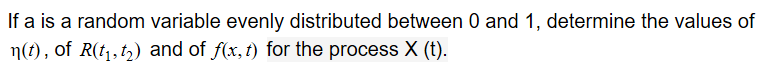Home / Answered Questions / Other / can-you-help-me-to-solve-one-of-these-questions-1-let-the-process-x-t-exp-at-be-a-family-of-exponent-aw923

# (Solved): Can You Help Me To Solve One Of These Questions? 1. Let The Process X (t) = Exp(at) Be A Family Of E...

Can you help me to solve one of these questions?

1. Let the process X (t) = exp(at) be a family of exponentials that depend on the random variable a. Express the mean Î· (t), the autocorrelation function R (t1, t2), and the first order density f (x, t) of X (t) in terms of the density fa (a) of the random variable a .

2.If a is a random variable evenly distributed between 0 and 1, determine the values of n(t), of R(t1, tz) and of f(x, t) for the process X (t).

We have an Answer from Expert